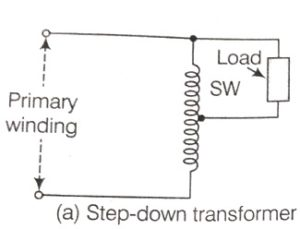# What is an Autotransformer?

An Autotransformer is a one winding transformer in which a part of the winding is common to both primary and secondary circuits.

The general construction and operating principle of an Autotransformer are the same as that of the conventional two winding transformers.

The Autotransformer differs from a conventional two winding transformer in a way that both the windings are interrelated.

In a conventional two winding transformer, the primary and the secondary windings are completely insulated from each other but are magnetically linked by a common core. Whereas in Autotransformer both the windings are connected electrically as well as magnetically.

The transfer of power in the case of a two winding transformer is only inductive transfer due to the transformer action. While in the case of Autotransformer total power transfer is due to inductive transfer and conductive transfer both.

The single continuous winding which is common to both primary and secondary is wound on a laminated silicon steel core and therefore both primary and secondary sections of this one winding are on the same magnetic circuit.

## Types of Autotransformer

If the whole winding works as a secondary winding and a section of the winding works as a primary winding, then it becomes a step-down transformer.Similarly, if the whole winding works as primary and a section of the winding works as the secondary winding, then it becomes a  step-up transformer.## AUTOTRANSFORMER EFFICIENCY

Autotransformers have higher efficiency than a conventional two-winding transformers. This is due to the  following reasons

1. In an ordinary transformer, the total electrical power is transferred from primary to secondary winding by the transformation which results in power loss.

Transformation Ratio:

The ratio of the number of turns in the secondary winding to the number of turns in the primary winding is called the transformation ratio. It is denoted by symbol K.

Transformation ratio(K)=Ns/Np=Es/Ep ….. (i)

Electric Power developed in the secondary(Ps) is equal to the electrical power developed in the primary(Pp).

Ps=Pp

Is×Es =Ip×Ep

Es/Ep =IP/IS ……   (ii)

From equation (i) and (ii)

 Transformation ratio(k) =Es/Ep =Ip/Is =Ns/Np

2. In an autotransformer, electrical power is transferred from primary to secondary partly by the process of transformation and partially by direct electrical connection and power transferred conductively produces no transformer loss.

1. It uses less winding material than a two winding transformer.

2. For the same output, An Autotransformer is smaller in size and cheaper than two winding transformers.

3.It has higher efficiency than an equivalent two winding transformer because due to the reduction in conductor and core materials the Ohmic losses in the conductor and the core losses are smaller.

4.voltage regulation of the Autotransformer is superior.

5.It has variable output voltage when a sliding contact is used for the secondary.

1. Larger short-circuit(fault) current as the effective per-unit impedance of an Autotransformer is smaller as compared to a two winding transformer which results in reduced internal impedance.

2. There is a direct connection between the high voltage side and the low voltage side. In the case of an open circuit in the common winding, the full primary voltage would be applied to the load on the secondary side. This high voltage may burn out or damage the equipment connected to the secondary side.

3. There is a loss of isolation between input and output circuits.

## APPLICATIONS OF  AUTOTRANSFORMER

1. It is used for starting induction motors and synchronous motors with a number of tappings.

2. It is used as variac(variable a.c) in the laboratory and other situations that require continuously variable voltage over wide ranges.

3. It is used for boosting supply voltage by a small amount in distribution systems to compensate for voltage drop.

4. It is used for the interconnection of power systems of different voltage levels.

5. It is used in automatic voltage stabilizers for domestic use or for servo stabilizers for industrial, commercial, and domestic use.

6. It is also used to tie together transmission or distribution circuits of slightly different voltages.

7.Autotransformer is also used as a furnace transformer for getting a conventional supply to suit the furnace winding from a 230v voltage supply.

VIEW MORE…